# Sparse Matrix and Polynomial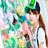3 de Feb de 2015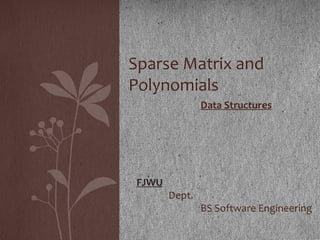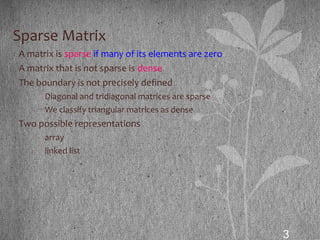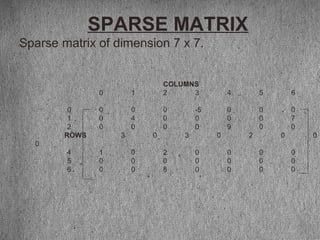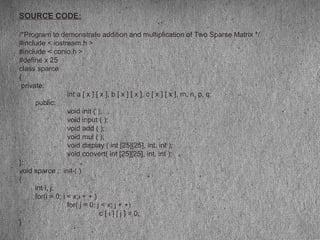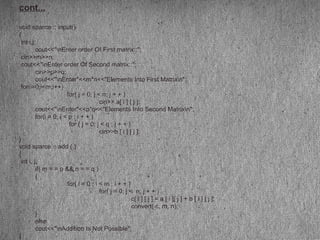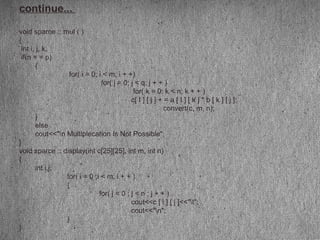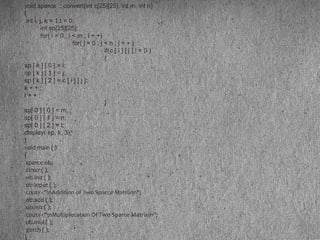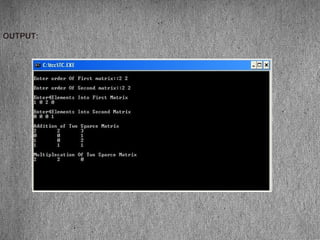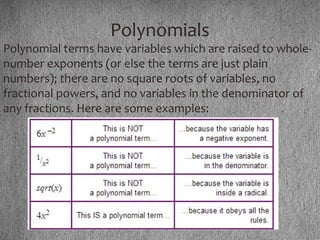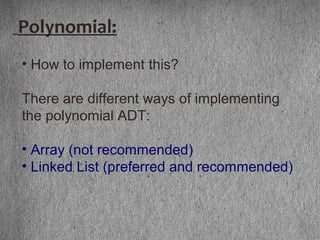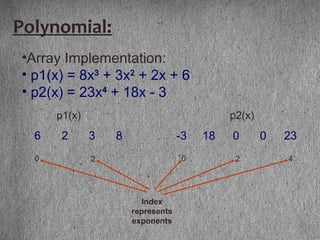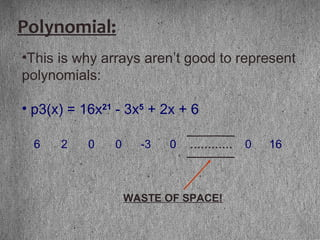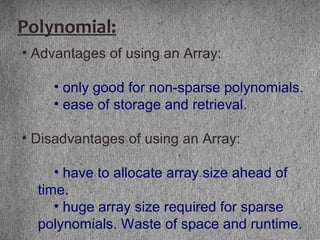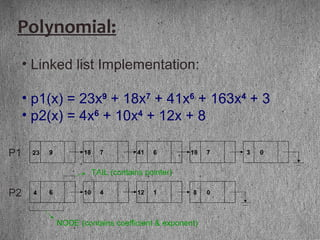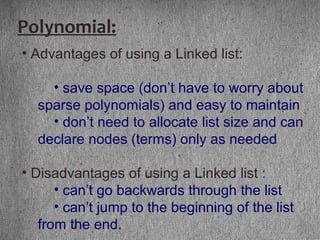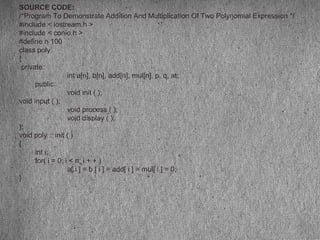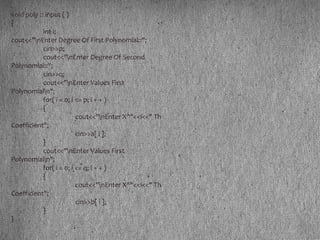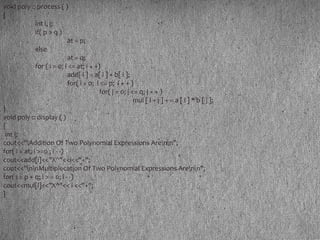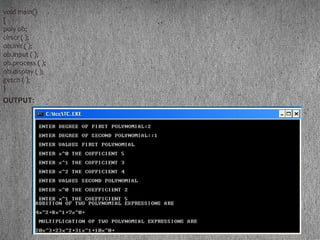1 de 20

### Sparse Matrix and Polynomial

• 1. Data Structures FJWU Dept. BS Software Engineering Sparse Matrix and Polynomials
• 3. 3 Sparse Matrix • A matrix is sparse if many of its elements are zero • A matrix that is not sparse is dense • The boundary is not precisely defined • Diagonal and tridiagonal matrices are sparse • We classify triangular matrices as dense • Two possible representations • array • linked list
• 4. SPARSE MATRIX Sparse matrix of dimension 7 x 7. COLUMNS 0 1 2 3 4 5 6 0 0 0 0 -5 0 0 0 1 0 4 0 0 0 0 7 2 0 0 0 0 9 0 0 ROWS 3 0 3 0 2 0 0 0 4 1 0 2 0 0 0 0 5 0 0 0 0 0 0 0 6 0 0 8 0 0 0 0
• 5. SOURCE CODE: /*Program to demonstrate addition and multiplication of Two Sparse Matrix */ #include < iostream.h > #include < conio.h > #define x 25 class sparce { private: int a [ x ] [ x ], b [ x ] [ x ], c [ x ] [ x ], m, n, p, q; public: void init ( ); void input ( ); void add ( ); void mul ( ); void display ( int , int, int ); void convert( int , int, int ); }; void sparce :: init ( ) { int i, j; for(i = 0; i < x;i + + ) for( j = 0; j < x; j + +) c [ i ] [ j ] = 0; }
• 6. cont... void sparce :: input() { int i,j; cout<<"nEnter order Of First matrix::"; cin>>m>>n; cout<<"nEnter order Of Second matrix::"; cin>>p>>q; cout<<"nEnter"<<m*n<<"Elements Into First Matrixn"; for(i=0;i<m;i++) for( j = 0; j < n; j + + ) cin>> a[ i ] [ j ]; cout<<"nEnter"<<p*q<<"Elements Into Second Matrixn"; for(i = 0; i < p ; i + + ) for ( j = 0; j < q ; j + + ) cin>>b [ i ] [ j ]; } void sparce :: add ( ) { int i, j; if( m = = p && n = = q ) { for( i = 0 ; i < m ; i + + ) for( j = 0; j < n; j + + ) c[ i ] [ j ] = a [ i ][ j ] + b [ i ] [ j ]; convert( c, m, n); } else cout<<"nAddition Is Not Possible"; }
• 7. continue... void sparce :: mul ( ) { int i, j, k; if(n = = p) { for( i = 0; i < m; i + +) for( j = 0; j < q; j + + ) for( k = 0; k < n; k + + ) c[ I ] [ j ] + = a [ I ] [ k ] * b [ k ] [ j ]; convert(c, m, n); } else cout<<"n Multiplecation Is Not Possible"; } void sparce :: display(int c, int m, int n) { int i,j; for( i = 0 ;i < m; i + + ) { for( j = 0 ; j < n ; j + + ) cout<<c [ i ] [ j ]<<"t"; cout<<"n"; } }
• 8. void sparce :: convert(int c, int m, int n) { int i, j, k = 1,t = 0; int sp; for( i = 0 ; i < m ; i + +) for( j = 0 ; j < n ; j + + ) if(c [ i ] [ j ] ! = 0 ) { sp [ k ] [ 0 ] = i; sp [ k ] [ 1 ] = j; sp [ k ] [ 2 ] = c [ i ] [ j ]; k + + ; t + + ; } sp[ 0 ] [ 0 ] = m; sp[ 0 ] [ 1 ] = n; sp[ 0 ] [ 2 ] = t; display( sp, k, 3); } void main ( ) { sparce ob; clrscr ( ); ob.init ( ); ob.input ( ); cout<<"nAddition of Two Sparce Matrixn"; ob.add ( ); ob.init ( ); cout<<"nMultiplecation Of Two Sparce Matrixn"; ob.mul ( ); getch ( ); }
• 10. Polynomials Polynomial terms have variables which are raised to whole- number exponents (or else the terms are just plain numbers); there are no square roots of variables, no fractional powers, and no variables in the denominator of any fractions. Here are some examples:
• 11. Polynomial: • How to implement this? There are different ways of implementing the polynomial ADT: • Array (not recommended) • Linked List (preferred and recommended)
• 12. Polynomial: •Array Implementation: • p1(x) = 8x3 + 3x2 + 2x + 6 • p2(x) = 23x4 + 18x - 3 6 2 3 8 0 2 Index represents exponents -3 18 0 0 23 0 42 p1(x) p2(x)
• 13. •This is why arrays aren’t good to represent polynomials: • p3(x) = 16x21 - 3x5 + 2x + 6 Polynomial: 6 2 0 0 -3 0 0 16………… WASTE OF SPACE!
• 14. • Advantages of using an Array: • only good for non-sparse polynomials. • ease of storage and retrieval. • Disadvantages of using an Array: • have to allocate array size ahead of time. • huge array size required for sparse polynomials. Waste of space and runtime. Polynomial:
• 15. • Linked list Implementation: • p1(x) = 23x9 + 18x7 + 41x6 + 163x4 + 3 • p2(x) = 4x6 + 10x4 + 12x + 8 23 9 18 7 41 6 18 7 3 0 4 6 10 4 12 1 8 0 P1 P2 NODE (contains coefficient & exponent) TAIL (contains pointer) Polynomial:
• 16. • Advantages of using a Linked list: • save space (don’t have to worry about sparse polynomials) and easy to maintain • don’t need to allocate list size and can declare nodes (terms) only as needed • Disadvantages of using a Linked list : • can’t go backwards through the list • can’t jump to the beginning of the list from the end. Polynomial:
• 17. SOURCE CODE: /*Program To Demonstrate Addition And Multiplication Of Two Polynomial Expression */ #include < iostream.h > #include < conio.h > #define n 100 class poly { private: int a[n], b[n], add[n], mul[n], p, q, at; public: void init ( ); void input ( ); void process ( ); void display ( ); }; void poly :: init ( ) { int i; for( i = 0; i < n; i + + ) a[ i ] = b [ i ] = add[ i ] = mul[ i ] = 0; }
• 18. void poly :: input ( ) { int i; cout<<"nEnter Degree Of First Polynomial::"; cin>>p; cout<<"nEnter Degree Of Second Polynomial::"; cin>>q; cout<<"nEnter Values First Polynomialn"; for( i = 0; i <= p; i + + ) { cout<<"nEnter X^"<<i<<" Th Coefficient"; cin>>a[ i ]; } cout<<"nEnter Values First Polynomialn"; for( i = 0; i <= q; i + + ) { cout<<"nEnter X^"<<i<<" Th Coefficient"; cin>>b[ i ]; } }
• 19. void poly :: process ( ) { int i, j; if( p > q ) at = p; else at = q; for ( i = 0; i <= at; i + +) add[ i ] = a[ i ] + b[ i ]; for( i = 0; i <= p; i + + ) for( j = 0; j <= q; j + + ) mul [ i + j ] + = a [ i ] * b [ j ]; } void poly :: display ( ) { int i; cout<<"Addition Of Two Polynomial Expressions Arenn"; for( i = at; i >=0 ; i - -) cout<<add[i]<<"X^"<<i<<"+"; cout<<"nnMultiplecation Of Two Polynomial Expressions Arenn"; for( i = p + q; i > = 0; i - -) cout<<mul[i]<<"X^"<< i <<"+"; }
• 20. void main() { poly ob; clrscr ( ); ob.init ( ); ob.input ( ); ob.process ( ); ob.display ( ); getch ( ); } OUTPUT: